Geometry

# Tangent and Secant Lines: Level 2 Challenges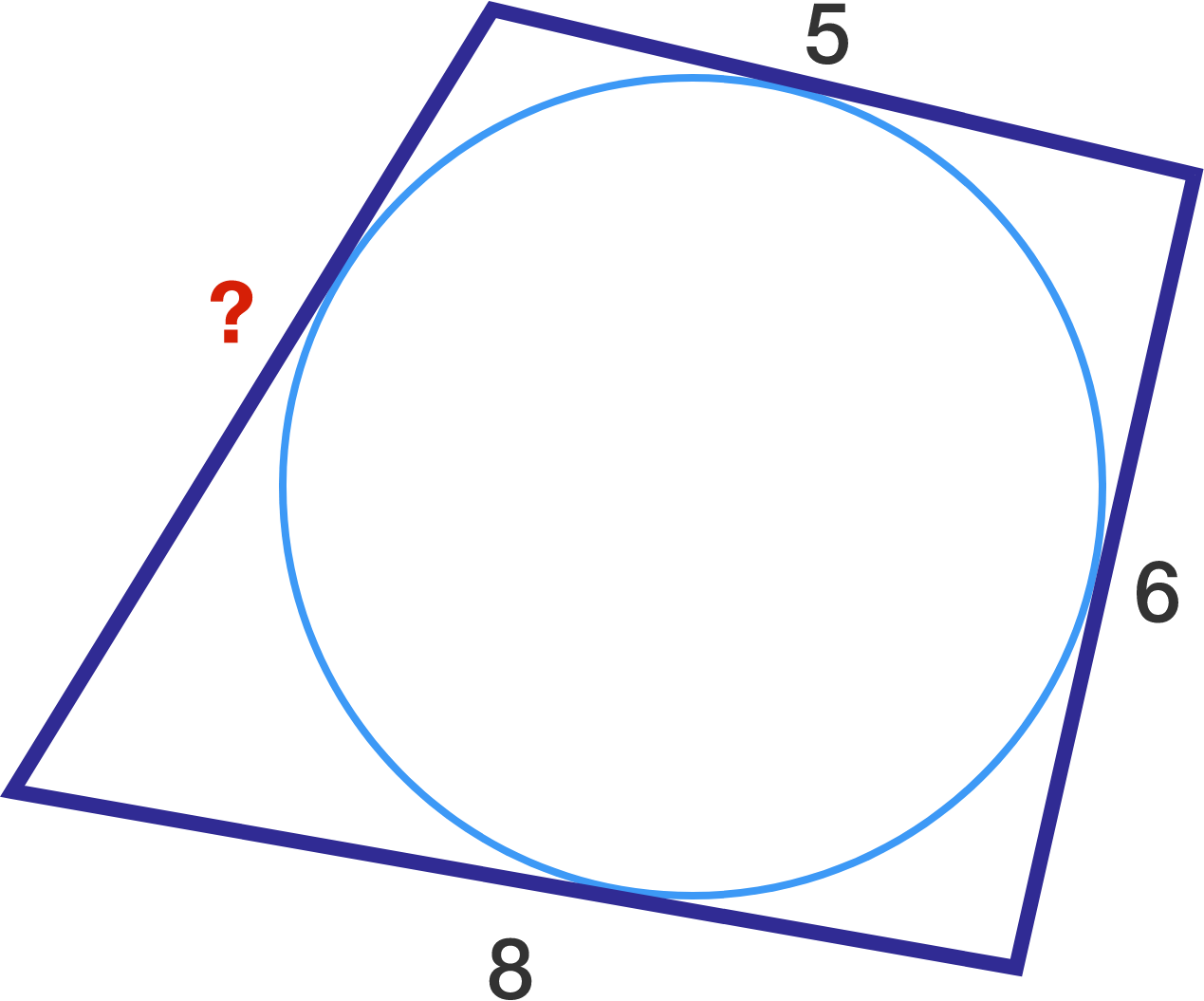Given the quadrilateral and inscribed circle, what is the missing side length?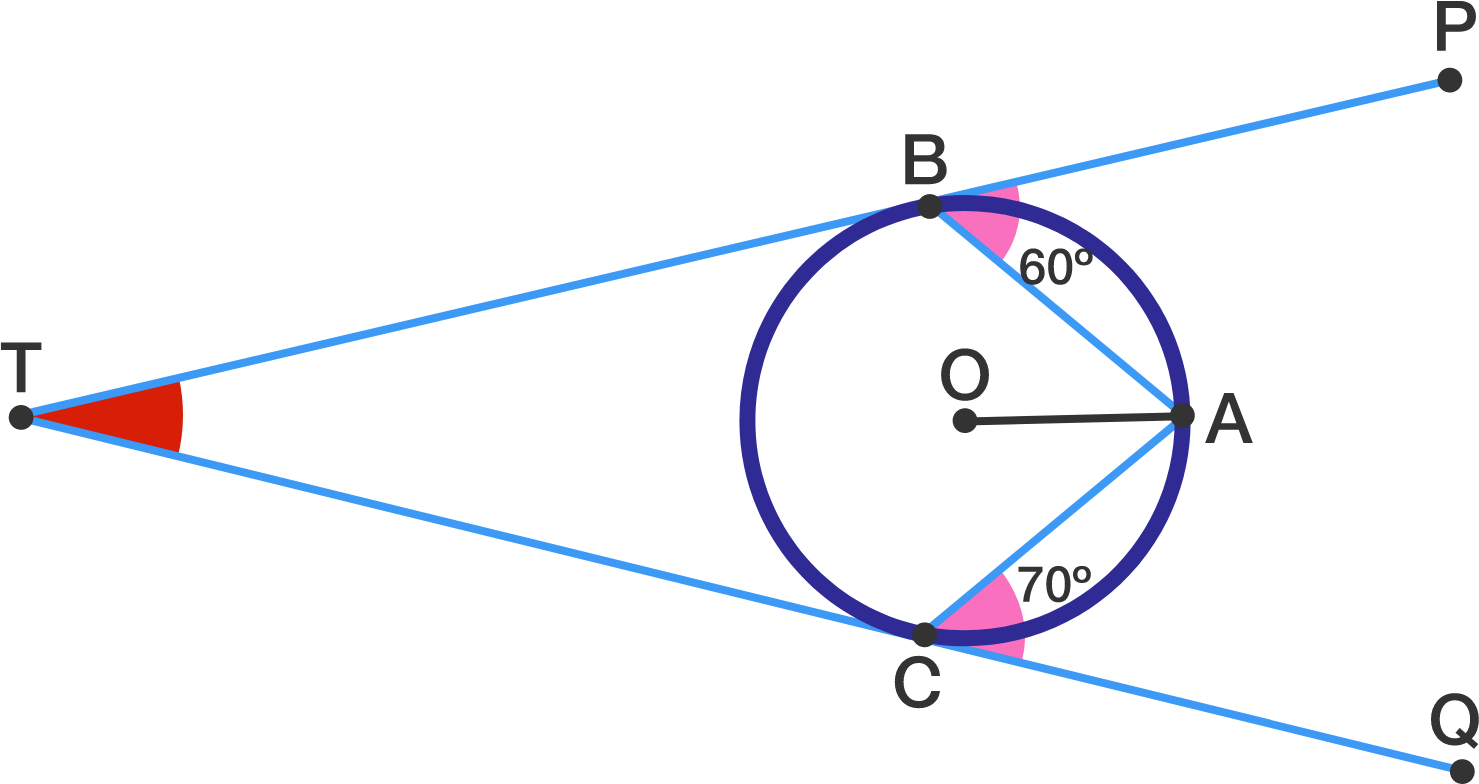In the figure given above $\overline{TP}$ and $\overline{TQ}$ are tangent to the circle with center $O$ at $B$ and $C,$ respectively.

If $\angle PBA=60^\circ$and $\angle ACQ=70^\circ$, what is $\angle BTC$ in degrees?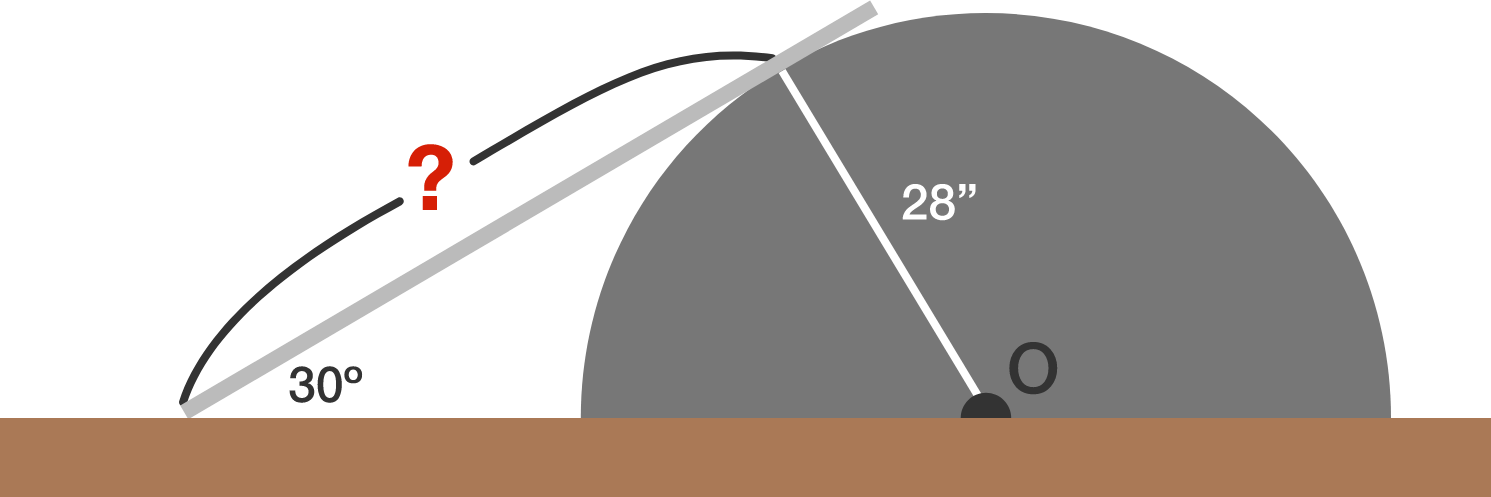There is a semi-circular object on a road and a strong metal plate is put on it, as shown in the above diagram, so that cars can better pass over it. If the radius of the object is $28$ inches and the angle between the plate and the road is $30^\circ,$ what is the distance (in inches) between the point the plate meets the road and the point the plate touches the semi-circular object?

A point $P$ is given outside of a circle $\Gamma$. A tangent from $P$ touches $\Gamma$ at $T$ with $PT=45$. A line from $P$ cuts $\Gamma$ at the 2 points $A, B$. If $PA=25$, what is the length of $PB$?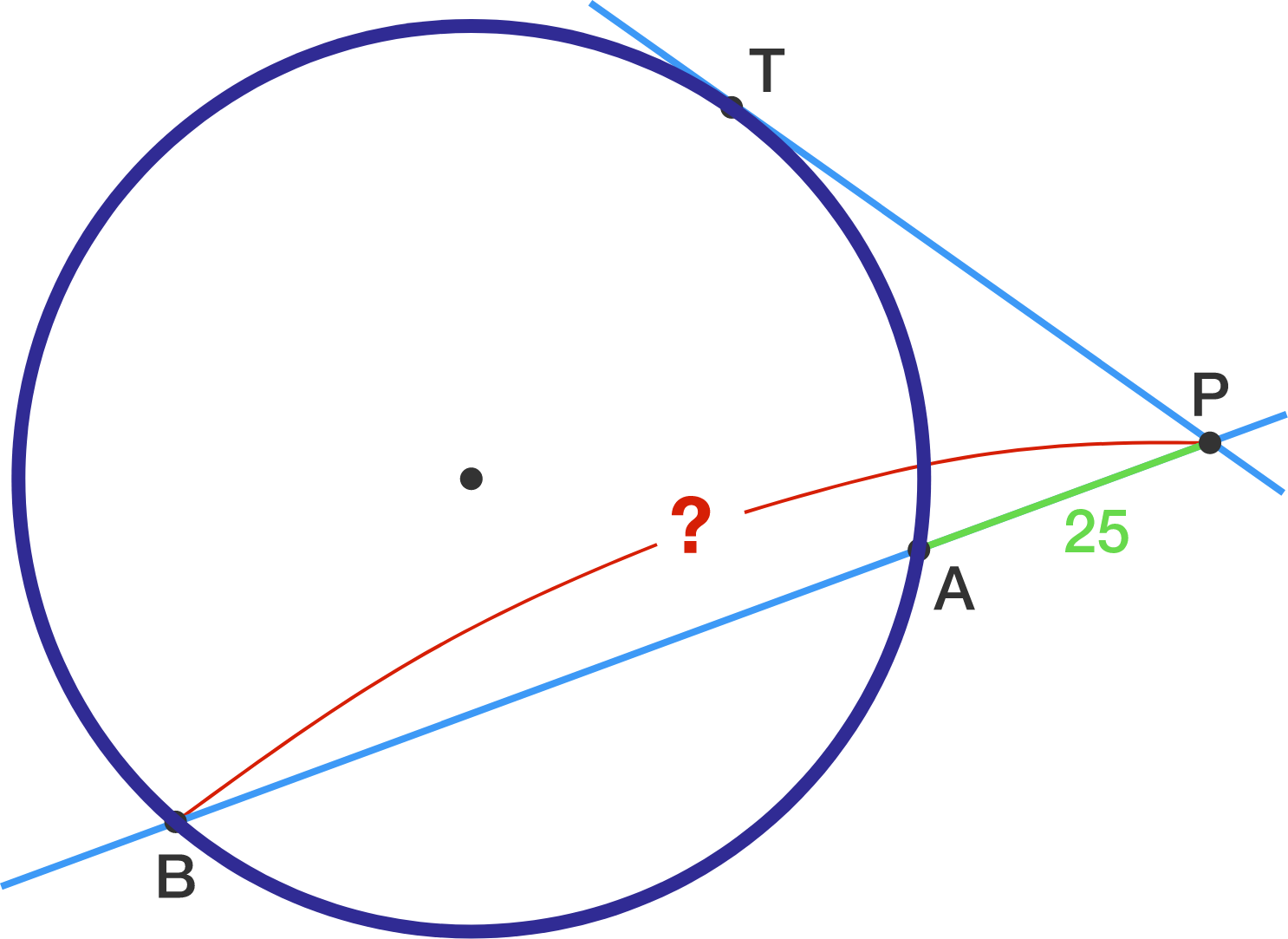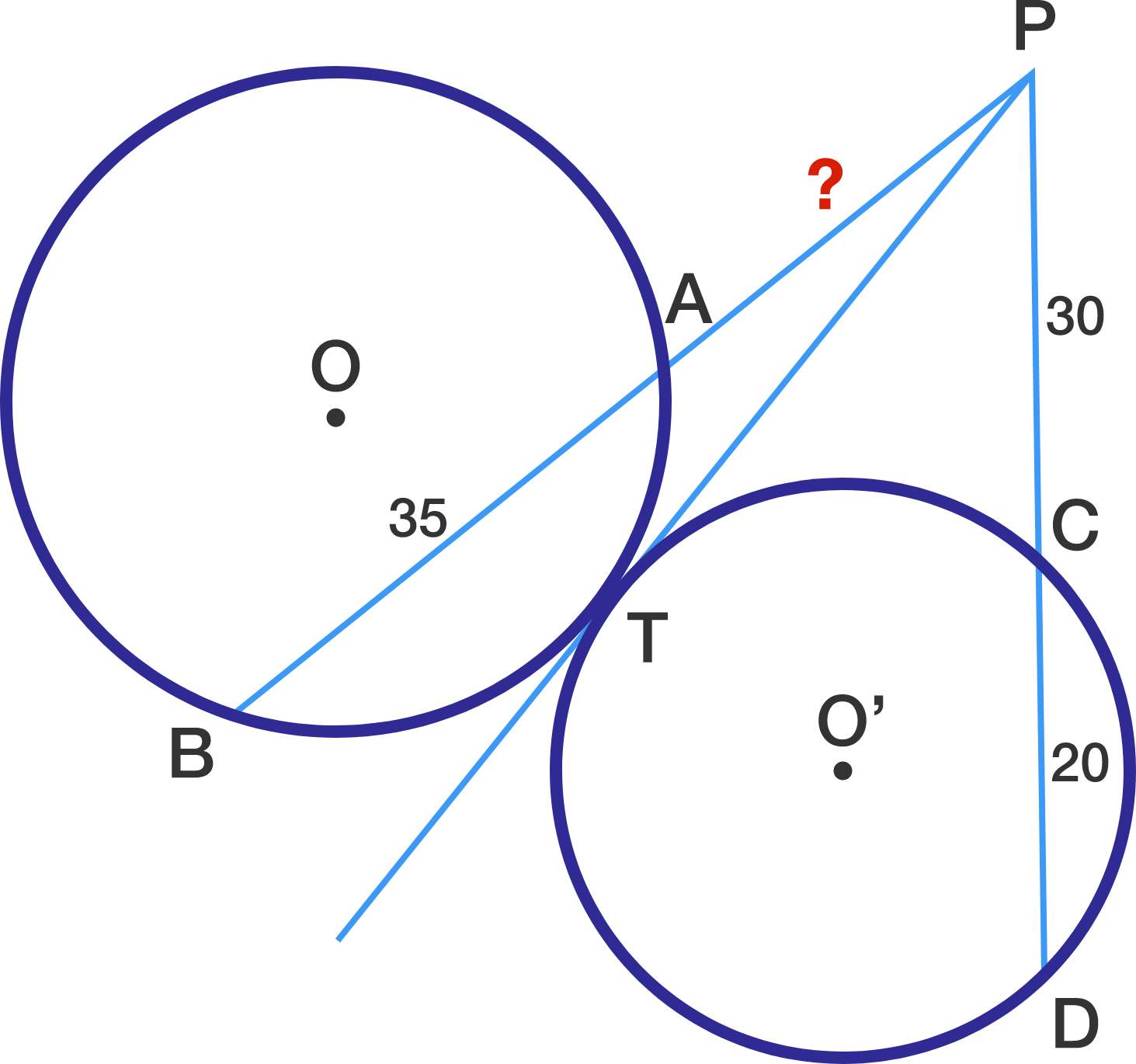In the above diagram, line segment $\overline{PT}$ is tangent to both circle $O$ and circle $O'.$ Given the following three lengths: $\lvert\overline{AB}\rvert = 35, \lvert\overline{PC}\rvert = 30, \lvert\overline{CD}\rvert = 20,$ what is $\lvert\overline{PA}\rvert?$

×